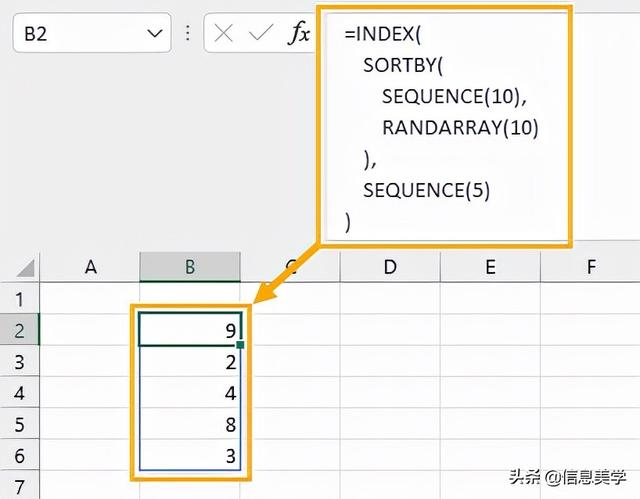Excel 中的随机数并非是每个用户都需要的功能 ，大多数人的数据都会使用固定数字和公式，可能不需要在他们的报告中出现随机数。

1/ 使用 RAND 函数生成随机数

Excel中有一个非常简单的RAND函数，它不需要参数，会生成一个 0 到 1 之间的随机数。

RAND 函数示例：

`= RAND ( )`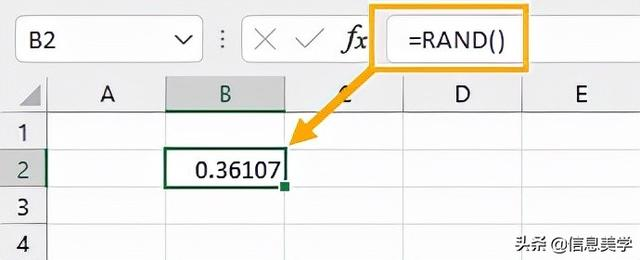`= RAND ( ) * ( Y - X ) + X`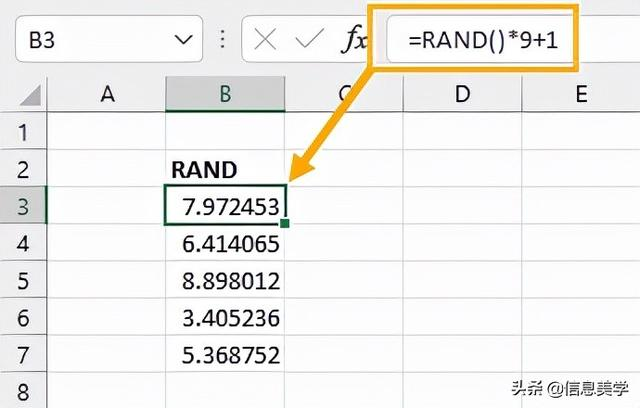`= ROUND ( RAND ( ) * ( Y - X ) + X, 0 )`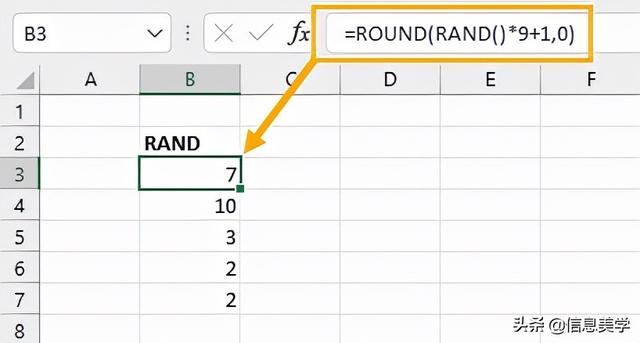`= ROUND ( RAND ( ) * ( 4 - -3 ) + -3, 0 )`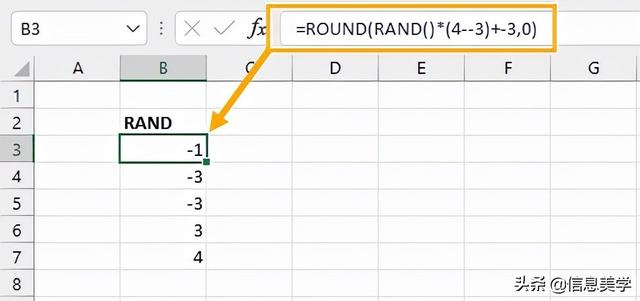2/ 使用 RANDBETWEEN 函数生成随机数

RANDBETWEEN 函数的语法：

`= RANDBETWEEN ( bottom, top )`

bottom 是返回值的下限top 返回值的上限 函数将在下限值和上限值之间生成随机整数

RANDBETWEEN 函数示例：

`= RANDBETWEEN ( -3, 4 )`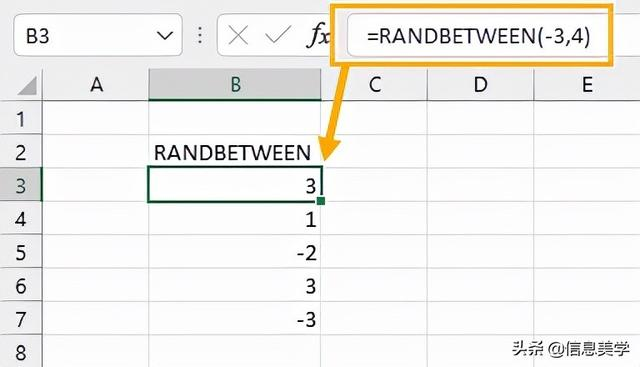3/ 使用 RANDARRAY 函数生成随机数

RANDARRAY 函数的语法：

`= RANDARRAY ( [rows], [columns], [min], [max], [whole_number] )`

rows是要返回的行数columns 是要返回的列数min 是随机数的最小值max是随机数的最大值whole_Number为 TRUE 返回整数，FALSE 返回小数

RANDARRAY 函数示例：

`= RANDARRAY ( 4, 3, 6, 14, TRUE )`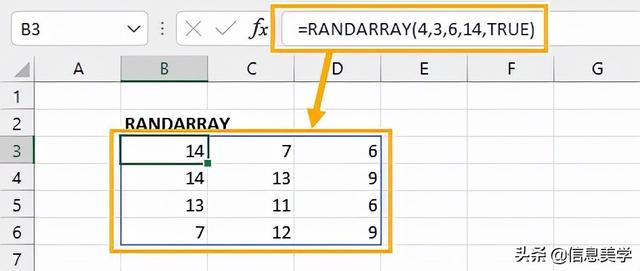4/ 使用分析工具插件生成随机数

1/ 单击功能区中的 文件 选项卡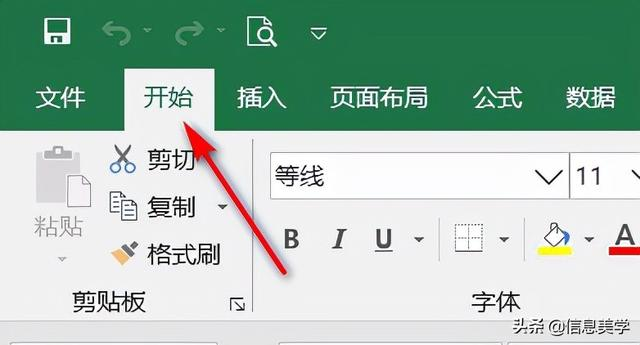2/ 在窗口的左下方窗格中，向下滚动并单击 选项3/ 在弹出窗口的左侧窗格中，单击加载项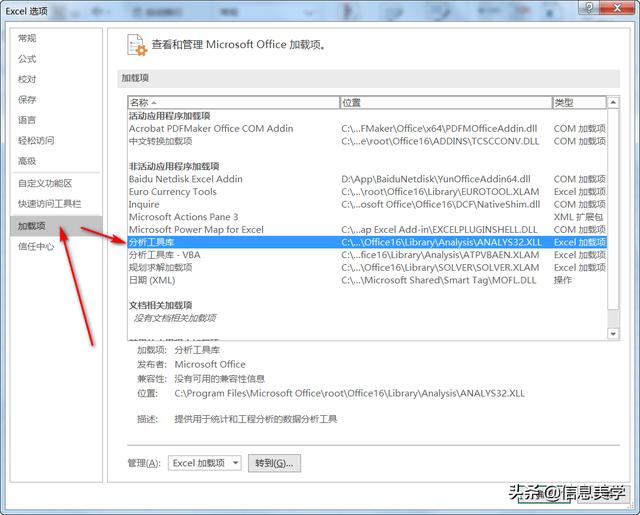4/ 勾选 分析工具库，单击确定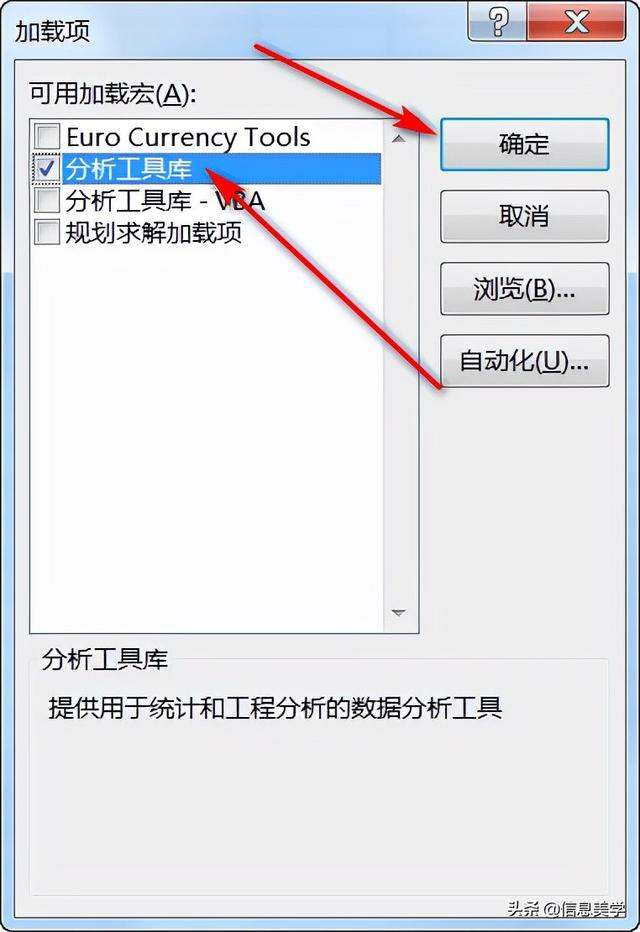5/ Excel 功能区的“数据”选项卡上，现在有一个名为“分析”的组，就可以看到到“数据分析”的选项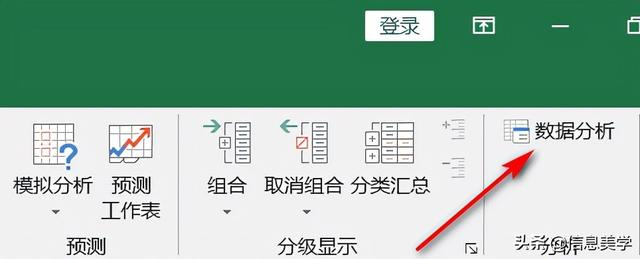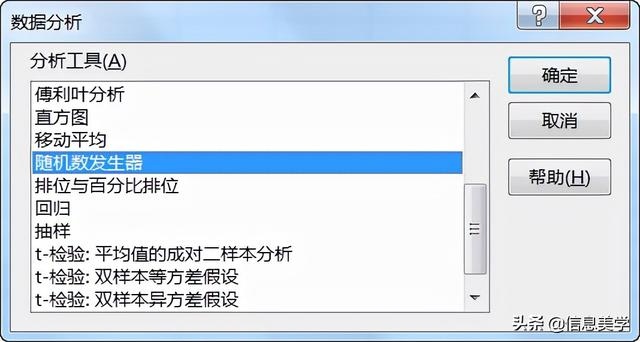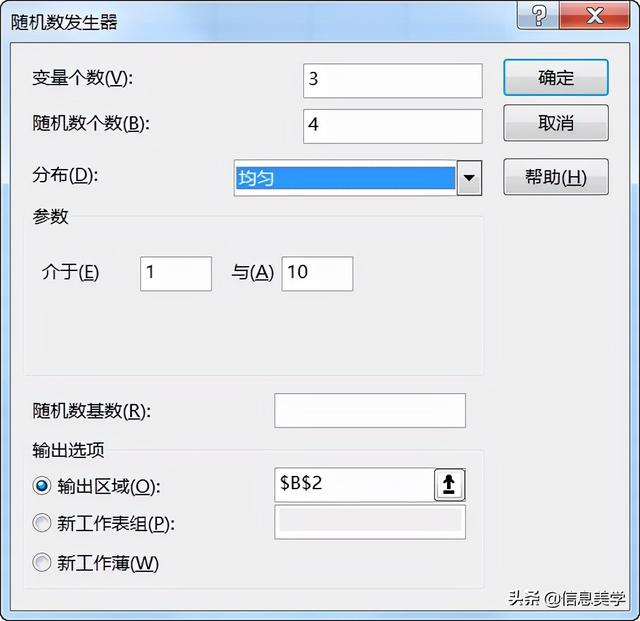5/ 用 VBA 生成随机数

VBA（Visual Basic for Applications）是位于 Excel 前端的编程语言，也可用于生成随机数。但是，它比简单地在 Excel 中的单元格中输入公式要复杂，而且需要一些编程知识才能使用它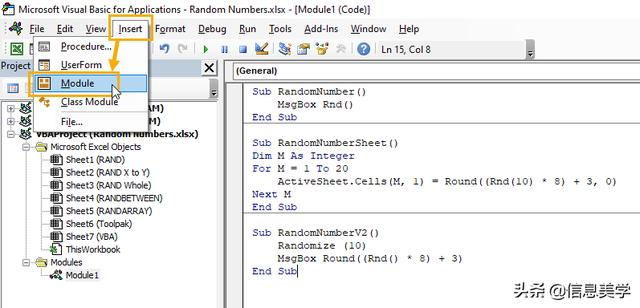`Sub RandomNumber() MsgBox Rnd()End Sub`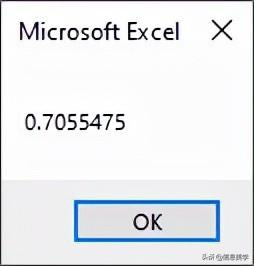`Sub RandomNumberSheet()Dim M As IntegerFor M = 1 To 5 ActiveSheet.Cells(M, 1) = Round((Rnd(10) * 7) + 3, 0)Next MEnd Sub`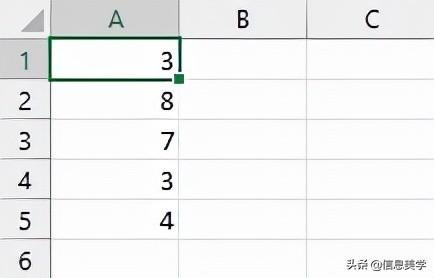`Sub RandomNumberV2() Randomize (10) MsgBox Round((Rnd() * 7) + 3)End Sub`

6/ 生成不重复的随机数

RANK.EQ 和 COUNTIF 函数的解决方案：

`= RANDBETWEEN ( 1, 10 )`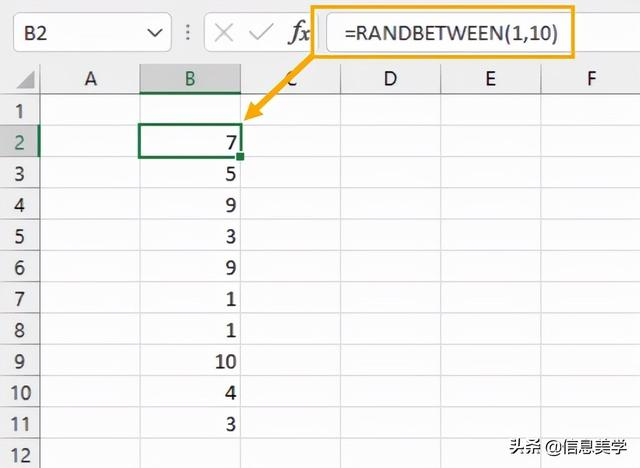`= RANK.EQ ( B2, \$B\$2:\$B\$11 ) + COUNTIF ( \$B\$2:B2, B2 ) - 1`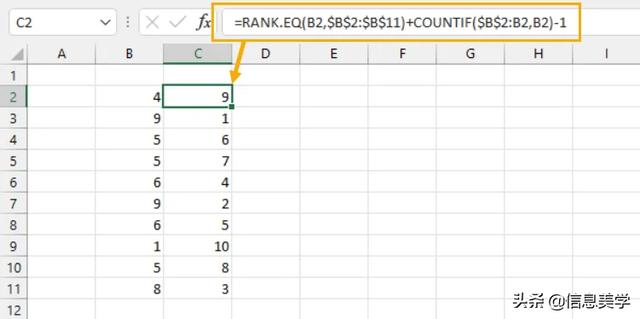`Sub RandomNumberNoDuplicates()Dim M As Integer, Temp As String, RandN As IntegerFor M = 1 To 5Repeat: RandN = Round((Rnd(10) * 9) + 1, 0) If InStr(Temp, RandN) Then GoTo Repeat ActiveSheet.Cells(M, 1) = RandN Temp = Temp & RandN & "|"Next MEnd Sub`

`=INDEX( SORTBY( SEQUENCE(10), RANDARRAY(10) ), SEQUENCE(5))`